The behaviour of the single value channels can be different depending on multiple factors (basic descriptions of each channel type can be found here).

In the examples below, we will use the same setup file with 3 formulas, but change the recalculation order: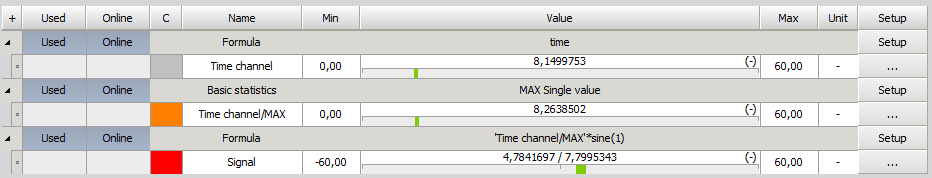Time channel - This is a synchronous time channel generated in formula. This channel will have the value of the elapsed time in the measurement. For example at 1 minute and 10 seconds the value of this channel will be 70.

Time channel/MAX - This is a single value basic statistics which will output the maximum of the time channel.

Signal - The signal channel is a synchronous 1 Hz sine wave which is multiplied by the single value channel.

Example no. 1 (All the channels are recalculated online during measurement):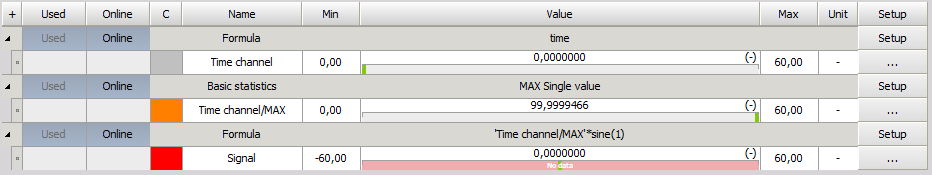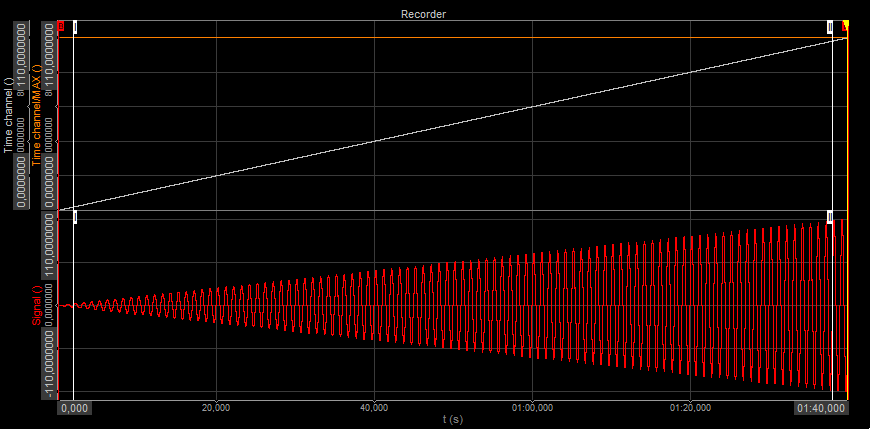Example no. 2 ("Signal" channel is set to offline and recalculated again):Example no.3 (Both, the single value basic statistics "Time channel/MAX" and the "Signal" channels are set to offline and recalculated again):The single value channels are calculated during the measurement similar as running basic statistics, but only the last value is saved. If the channels are used in synchronous mathematics, the mathematics will always take the current value of the channel, that is also why in the example 1 and 3, the amplitude of the "Signal" is not constant.

In example 2, the single value channel is already recalculated, so the mathematics get the same value no matter in which part of the file it is being calculated. The amplitude of the "Signal" is the same through the whole measurement.

Bellow are some additional examples where the changes can be also seen on the digital meter.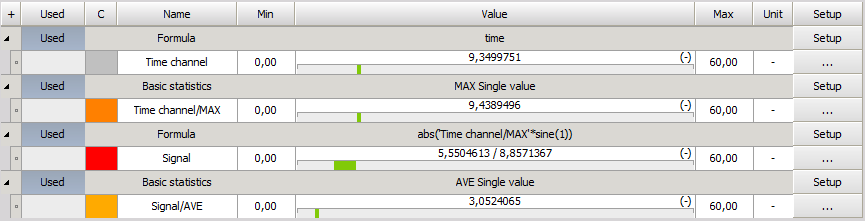We have changed "Signal" and added "Signal/AVE":

Signal - The signal channel is a synchronous 1 Hz sine wave which is multiplied by the single value channel. The formula has additional absolute function, so the signal shows absolute values of the Sine wave.

Signal/AVE - This if a single formula which outputs the average of the "Signal".

Example no. 1 (All the channels are recalculated online during measurement):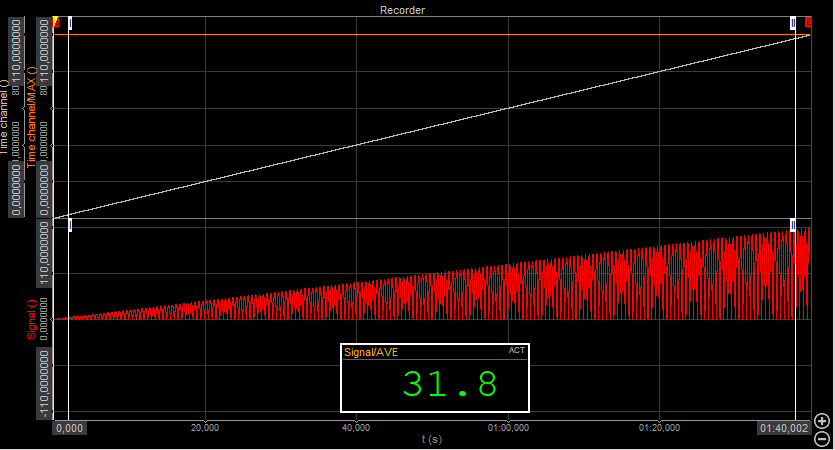Example no. 2 ("Signal" and "Signal/AVE" channels are set to offline and recalculated again):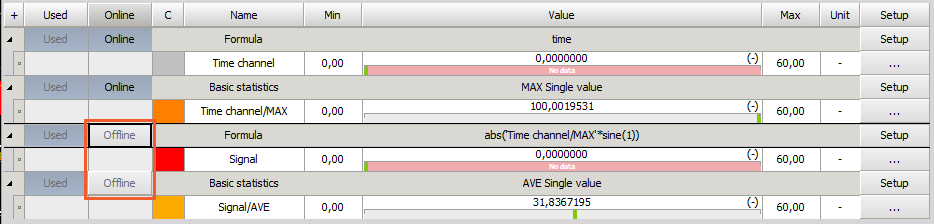Example no.3 (The "Time channel/MAX", "Signal" and "Signal/AVE" channels channels are set to offline and recalculated again):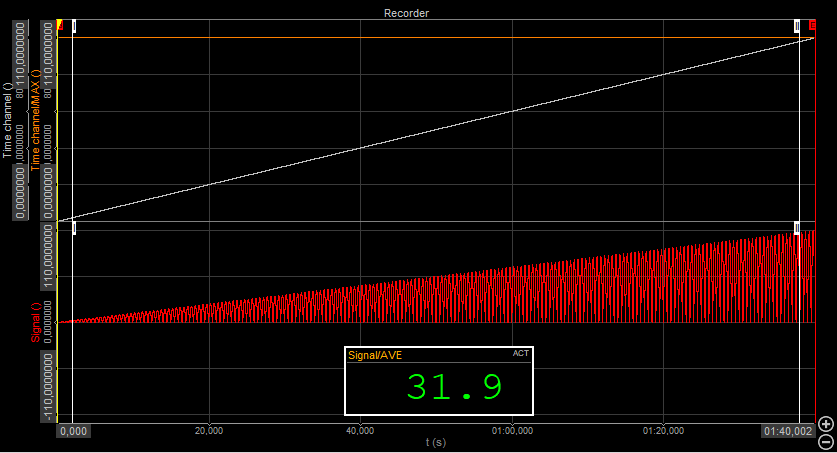When the single value "Signal/AVE is recalculated during the measurement the absolute sine amplitude increases from 0 - 100 but when the "signal" and "Signal/AVE" channels are recalculated separately, the amplitude of the absolute sine is always 100. Because of the change of the "Signal" channel, the single value average is different.

In the example no. 3, we get the same result as during the online calculation because all the channels are recalculated simultaneously (same as online).